# Ordinary fractions

## Definition. Elements of the fraction. Classification fractions

### Definition.

An ordinary fraction is a pair (a, b) of two natural numbers a and b, with b different by 0. The ordinary fraction (a, b) is writtenor a/b.

Examples of fractions: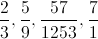A fraction (in Latin we have: fractus, i.e. "broken") represents a part of a whole or, any number of equal parts. When spoken in everyday English, a fraction describes how many parts of a certain size there are, for example, one-half, seven-fifths, three-quarters.

### The elements of a fraction

In this fraction, a is numerator and b is the denominator. Just like this example: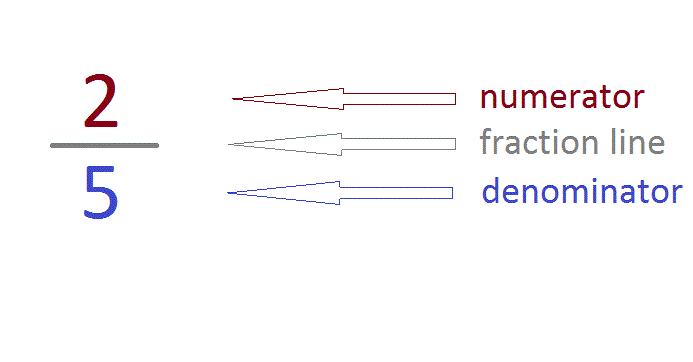We read two fifths.

Any of natural numbers can be written as a fraction. e.g.,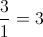### Classification fractions

A fraction the denominator is less than the numerator is called fraction below par. This is a proper fraction.

For example,is a fraction below par.

A fraction with the numerator equal to the fraction denominator is called improper function. Also the fractions where the numerator is bigger than the denominator.

For example,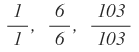,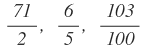### Comparing fractions

Two fractions are equivalent fractions when they represent the same part of a whole.

Two fractions are equal if the following condition is satisfied: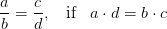Of the two fractions with the same denominator is greater the fraction with greater numerator.

For example,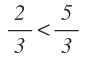,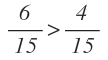Of the two fractions with the same numerator is higher fraction with smaller denominator.

For example,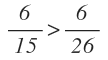,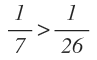When we want to compare two fractions that not have denominators equal or neither even numerator can bring fractions to the same denominator and apply the above rules.

Or apply the following rule: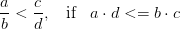Keywords: arithmetic, fractions, fractions ordinary lessons

### Forum

Here you can discuss about mathematic, about algebra, geometry, trigonometry.

It is not mandatory to be logged in on this forum but it is nice to have an account. You can ask about mathematics just with your name and your email.

This maths forum is one of the easiest forums to use it.

>> Go to Math Forum